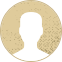Top Special Offer! Check discount

Get 13% off your first order - useTopStart13discount code now!

# Analysis of Variance

132 views 2 pages ~ 342 words
Get a Custom Essay Writer Just For You!

Experts in this subject field are ready to write an original essay following your instructions to the dot!

[Author Name(s), First M. Last, Titles and Degrees Omitted]

[Affiliation(s) with an Institution]

Variance Analysis (ANOVA)

Problem: The mean income level of three states, Alaska, Michigan, and Seattle, is used to investigate the occurrence of any paygrade differences. Five companies with branch offices in all three states were chosen, and the median yearly income of these companies was collected, as shown below:

The Company's Name

Michigan

Seattle

33.568 48.273 57.37 Company A

30.505 48.1 55.66 Company B

33.156 48.6 61.42 Company C

31.205 46.2 56.246 Company D

E Company 28.9 35.056 51.245

*Figures are in Thousands

Null Hypothesis:

There is no significant difference among the median salaries in these states.

Mathematically, H0: μ1 = μ2 = μ3

Alternative Hypothesis:

At least one state has a different paygrade among these three states.

Mathematically, H0: μ1 ≠μ2 ≠ μ3

Let us perform an ANOVA test to testify the hypothesis by analyzing the variances in each category.

Step 1: The first step consists of computing variance for each group. It can be done by using excel function VAR.S () as follows:

Step 2: The Mean Square Error (MSE) is given by the mean of all variances above.

MSE = = 16.79

Step 3: Now compute the variance of three variances computed above. The variance is:

228.24

The Mean Square Between (MSB) value can be obtained by multiplying the above variance by the number of companies, which is 5 in this case. MSB = 1141.21

Step 4: F-value may be obtained from the quotient of MSB and MSE.

F-value = = = 13.59

Step 5: degrees of freedom, df1 and df2 may be computed as follows. We have three different categories (states) with 5 number of companies in each. This gives us,

df1 = 3-1 = 2 and

df2 = 3× (5-1) = 3×4 =12

Step 6: p-value may be obtained from Excel function F. DIST.RT(F-value, df1, df2). Here the degree of freedoms, df1 and df2 are 2 and 12 respectively.

p-value = 0.00033329

Step 7: Let us consider a 5% level of significance for which α = 0.05. If the p-value is less than the level of significance then we reject the null hypothesis and accept otherwise. Here, p-value of 0.00033329 is much less than 0.05; we reject the null hypothesis.

Step 8: Decision: The ANOVA test confirms the presence of significant amount of difference among the income level of these states.

April 06, 2023
Category:
Subject area:
Number of pages

2

Number of words

342

32Rate:

4.6

Expertise Company
Verified writer

JakeS has helped me with my economics assignment. I needed an urgent paper dealing with Brexit. JakeS has been awesome by offering an outline with ten sources that have been used. It helped me to avoid plagiarism and learn more about the subject.

Hire Writer

This sample could have been used by your fellow student... Get your own unique essay on any topic and submit it by the deadline.

Eliminate the stress of Research and Writing!

Hire one of our experts to create a completely original paper even in 3 hours!